E58 Control Theory: Lab 3
Proportional and Integral Control of a DC Motor
in partnership with aron dobos, david luong and mark piper
February 10, 2005

Abstract

In this laboratory experiment, a DC motor was controlled by proportional and integral controllers.Ā After the motor was allowed to spin up to steady state, a load motor was enabled.Ā The proportional controller was not able to recover from the perturbation, while the integral controller was able to. ĀThe integral controller also achieved a zero error signal, while the proportional controller could not because then the motor would have stopped rotating. The simulated responses of the system to the step inputs were calculated in MATLAB and compared to the experimental data.Ā The experimental and theoretical results compared very favorably, confirming the accurate characterization of the motor system in Lab 2.

Transfer Functions of the Motor System

The motor system is given in the following block diagram.Ā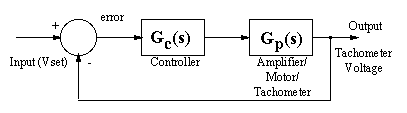For the proportional controller, the motor system transfer function, where GC = kp is given byFor integral control, the motor system transfer function isGraphs of Experimental Data

The theoretical responses shown in the graphs below were calculated in MATLAB using the tf and step commands.Ā The disturbance by shorting the load motor wires was applied approximately in the middle of the sampling time, allowing the motor to achieve steady state again after the disturbance.

Proportional Control

Graph 2A. Proportional kp=0.75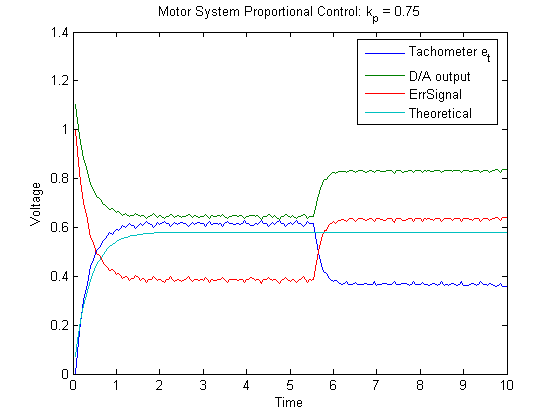Graph 2B. Proportional kp=1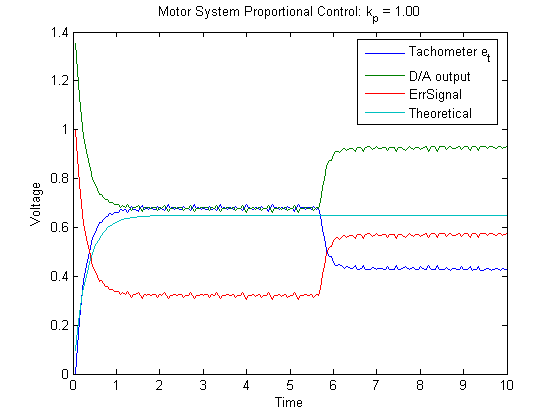Graph 2C. Proportional kp=2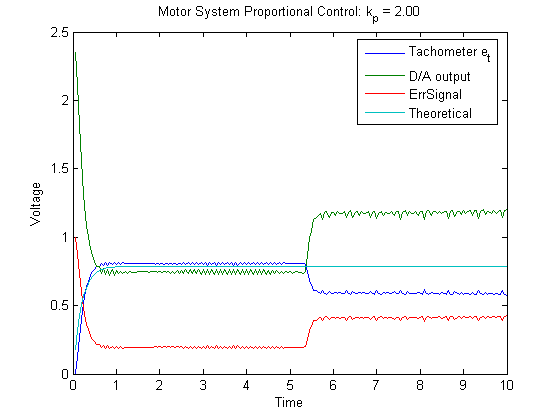Graph 2D. Proportional kp=5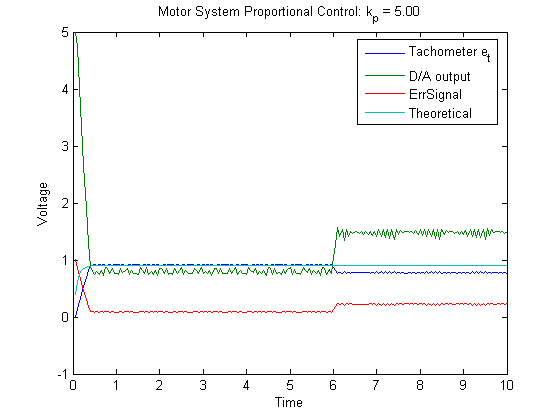Integral Control

Graph 2E. Integral ki=0.067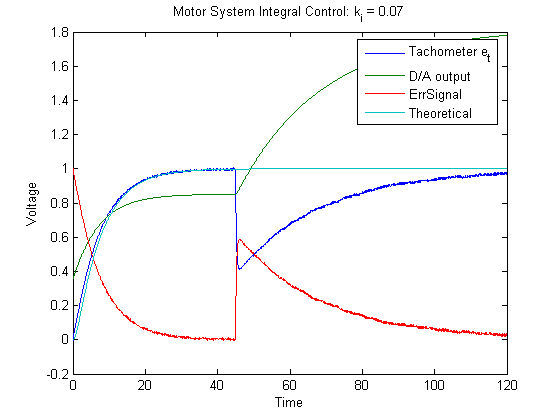Graph 2F. Integral ki=0.151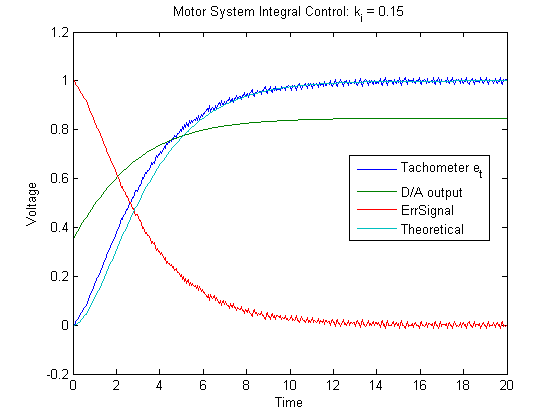Graph 2G. Integral ki=0.302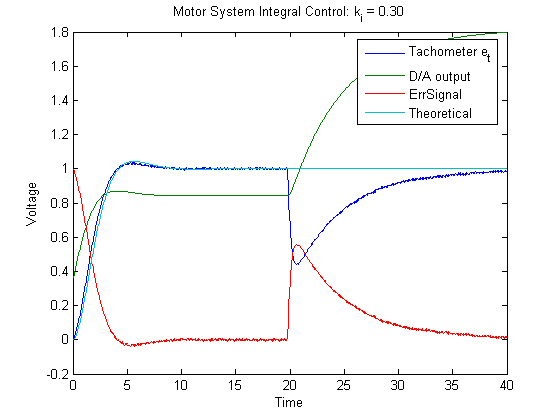Graph 2H. Integral ki=1.677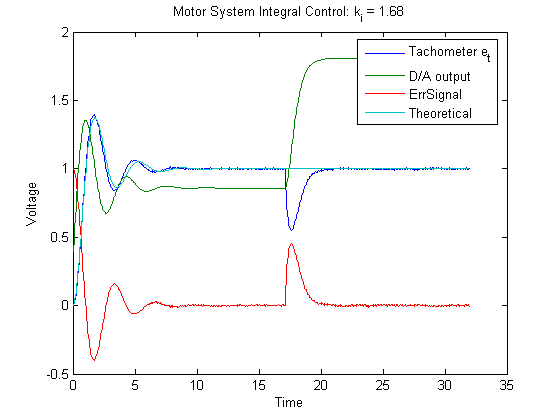Graph 2I. Integral ki=3.773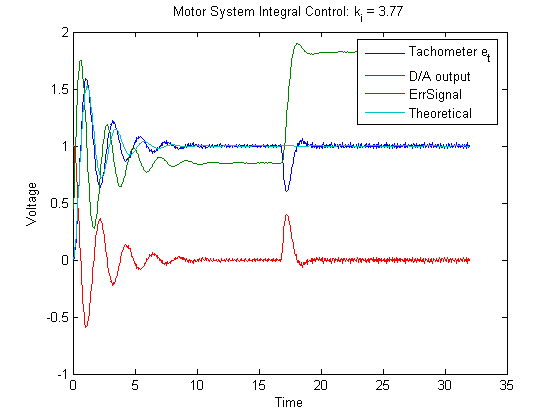Steady State and Time Constant for Proportional Control

For the proportionally controlled motor system, the time constant and steady state (sÓ0) for a unit step values are found by inspection of the transfer function given previously.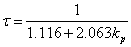Ā,Ā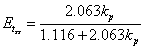Various time constants and steady state values are given in Table 4A below.

Table 4A. Theoretical and Experimental Time Constants and Steady State Values.

 Proportionality Constant () Time Constant (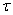) Steady State Value Theoretical Experimental Theoretical Experimental 0.75 0.375 0.37 0.581 0.614 1 0.314 0.30 0.649 0.678 2 0.191 0.25* 0.787 0.797 5 0.087 0.27* 0.902 0.897

* The experimental response no longer is in the shape of a normal exponential because the motor is ramped up too quickly by the large kp values.

Damping Ratio and Natural Frequency for Integral Control

For the integral controller, the 2nd order damping ratio and natural frequency are given by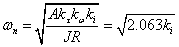Ā,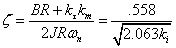To calculate experimental values of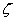and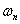, we use the equations given below.Ā We define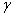Āas the percent overshoot.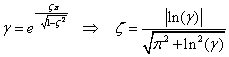Looking at the graphs, we can calculate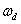Āfrom measuring the period of damped oscillation on the graphs.Ā We use the following equation to obtain the natural frequency.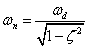Table 5A. Theoretical and Experimental Damping Ratios and Natural Frequencies

 Proportionality Constant (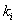) Damping Ratio () Natural Frequency () Theoretical Experimental Theoretical Experimental .067 1.501 N/A* 0.372 N/A* .151 0.999 N/A* 0.558 N/A* .302 0.707 0.738 0.789 0.983 1.677 0.299 0.287 1.860 2.150 3.773 0.200 0.164 2.789 2.962

* For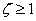, the characteristic equation of the transfer function factors into two real roots, so there is no oscillation.Ā As a result, the damping ratioĀand natural frequencyĀdo not apply.

Extension

We created a PID controller in simulink as shown in F1 below.Ā For the purposes of this lab, we set d to zero to create a PI controller and explored the optimal system response which we define to be when=.707.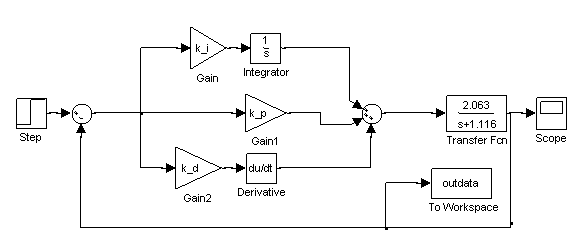Figure F1. Simulink block diagram for a PID controller.

The characteristic equation of the system with a PI controller in place can be shown to be: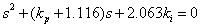Imposing our constraint of=.707 reveals the following relationship between ki and kp: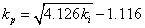Choosing a few particular values for kŁŁI and kp yields the results shown in Figure F2 below.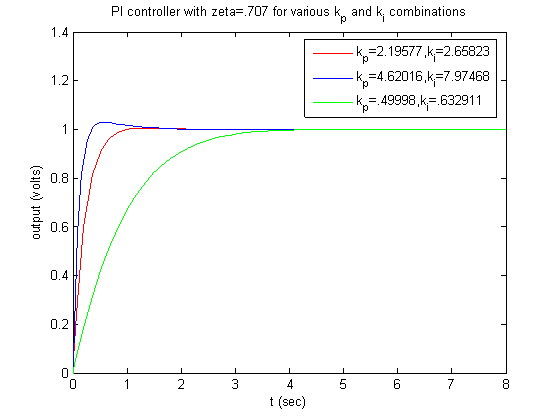Figure F2. PI controller system response with=.707 for selected kp and ki.

Depending on the value of ki, the natural frequency of the system changes, making the system faster or slower.Ā Increasing the system speed by choosing a higher value of ki comes at the expense of greater overshoot as seen in Figure F2.Ā The ideal balance of system speed and overshoot is dependent on the particular application of the system.

Points of Interest / Conclusion

From the lab, the basic difference we found for the two different controllers is the impact each has on the steady state error of the system response.Ā As shown from graphs 2A-2D, the steady state error is not zero corresponding to the proportional control system.Ā Graphs 2E-2I, corresponding to the integral control, clearly shows that the system steady state error approaches zero.Ā The fundamental difference between the two controllers is that the integral control integrates the steady state error, thus giving the system a non-zero error input to drive the system.Ā Having the system driven serves to allow it to steadily reduce the error until it is zero.Ā Without the integrating term (effectively having a proportional control), the steady state error can be non-zero as mentioned.

The integral controller also added a new response feature to the system by making the system response second order.Ā Depending on the value of, the value ofof the system is determined.Ā Whenless than 1, the system response is underdamped and exhibits a decaying oscillation that approaches the final value.Ā Whenis greater than 1, the system is overdamped with a response that has no overshoot approaching the final value.Āequals 1 is the case of critical damping when the response approaches the final value the quickest with no oscillations.Ā The system error displays the same response characteristic shown in graphs 2E-2I.

The motor was shorted out during the latter part of each trial run by pulling a switch, and the responses are shown in the graphs found in section 2.Ā As expected, the proportional control system reaches its new steady state value with the addition of the load, but the steady state error does not approach zero.Ā In contrast, the steady state error of the integral control system approaches zero.

For the proportional control, when the k values were too high (k > 1) the D/A output ramped, thus causing the tachometer voltage to do the same.Ā The theoretical and experimental system responses are no longer similar to one another. (Refer to Graph 2C and 2D)

The theoretical system response of the system had the effects of Coulombic friction included, and matched the experimental responses quite well as shown in the graphs.Ā The time constants, damping ratios, natural frequencies, and steady state values matched quite well as shown in Tables 5A and 4A.Ā

The coupling component on the motor shaft snapped during the middle of the lab.Ā We replaced the malfunctioned part with one from another apparatus, and continued the lab.Ā The fix should not greatly affect the performance of the motor and thus our results.Ā

We had to be careful with our desired voltage outputs; since the bipolar operational power supply / amplifier cannot output a voltage greater than 36 volts (or ~6 Amps) we maintained a low desired output voltage to ensure we did not saturate the amplifier.

MATLAB SCRIPTS FOR INTEGRAL CONTROLLER

function [] = integral(k_i, SampleTime)
% runs the motor, plots some graphs, and saves some data
% usage: intergral(k_i, SampleTime)
%

% Engineering 58 Lab 3

% David Luong, Mark Piper, akader, Aron Dobos

% Set up sampling parameters

ĀĀĀĀĀĀĀĀĀĀĀĀ
%Clear the display
SampleFreq
=20;ĀĀĀĀĀĀĀĀ %Sampling Frequency
%SampleTime=25;ĀĀĀĀĀĀĀĀĀĀ %sampling time (seconds)

if
SampleTime*SampleFreq >= 2000
ĀĀĀ
return
end

NumPts
=SampleFreq*SampleTime;ĀĀĀĀĀĀĀĀĀĀĀĀ %Number of points in run (<=2000).
PreSample
=0;ĀĀĀĀĀĀĀĀĀĀ %Number of points before control
ĀĀĀĀĀĀĀĀĀĀĀĀĀĀĀĀĀĀĀĀĀĀĀ
%Ā algorithm runs (this generates
ĀĀĀĀĀĀĀĀĀ ĀĀĀĀĀĀĀĀĀĀĀĀĀĀ
%Ā baseline data);
InChan
=0;ĀĀĀĀĀĀĀĀĀĀĀĀĀĀ %Input channel to use on DAQ board
OutChan
=0;ĀĀĀĀĀĀĀĀĀĀĀĀĀ %Output channel to use on DAQ board
DT=1/SampleFreq;ĀĀĀĀĀĀĀ
%Sampling Interval
%Setup the input channel

%You shouldn't need to change this.

Ain
Ain.SampleRate
=SampleFreq;
Ain.SamplesPerTrigger
=NumPts;
Ain.InputType
='SingleEnded';
Ain.BufferingConfig
=[1Ā 2000];
Ain.TransferMode
='Interrupts';
(Ain,InChan);
%Setup the output channel

%You shouldn't need to change this.

Aout
= analogoutput('nidaq');
(Aout,OutChan);

%%%%%%%%%%%%%%%%%%%%%%%%%%%%%%%%%%%%%%%%%%%%%%%%%%%%%%%%%%%

%%%%%%%% Predefine any arrays to make loop quicker %%%%%%%%

%%%%%%%%%%%%%%%%%%%%% Change as needed %%%%%%%%%%%%%%%%%%%%

%%%%%%%%%%%%%%%%%%%%%%%%%%%%%%%%%%%%%%%%%%%%%%%%%%%%%%%%%%%

TachData
=zeros(NumPts,1);
=zeros(NumPts,1);
ErrSig
=zeros(NumPts, 1);
%%%%%%%%%%%%%%%%%%%%%%%%%%%%%%%%%%%%%%%%%%%%%%%%%%%%%%%%%%%

%Define Time Vector (time=0 after PreSample is done)

t=((1:NumPts)-PreSample)*DT;

LastIndex
=0;
start(
Ain);

% turn off the motor initially - the controller will turn it on as

% necessary

putsample
(Aout,0);Ā

% apply a step input to the motor.Ā This applies 2.5*7.2 = 18 V

integrator
= 0;

% integrator controller constant

%k_i = 3.773;

% this is the desired tachometer voltage (e_t, the output)

DesiredTachVoltage
= 1;
while
(Ain.SamplesAcquired<NumPts),
ĀĀĀ j=Ain.SamplesAcquired;

ĀĀĀ
while(LastIndex==j),ĀĀĀĀĀĀĀĀĀĀĀ %Wait for next datum.
ĀĀĀĀĀĀĀ j=Ain.SamplesAcquired;

ĀĀĀ
end
ĀĀĀ
if ((j-LastIndex)~=1),ĀĀĀĀĀĀĀĀĀ %Make sure no data was missed.
ĀĀĀĀĀĀĀ disp(sprintf(
'Warning, datum skipped: %d, %d',LastIndex,j));
ĀĀĀ
end
ĀĀĀ InData=peekdata(Ain,j);ĀĀĀĀĀĀĀĀ
%Retrieve most recent datum.
ĀĀĀ
if (t(j)<0),ĀĀĀĀĀĀĀ %If during PreSample, output 0.
ĀĀĀĀĀĀĀ TachData(j)=0;

ĀĀĀ
elseĀĀĀĀĀĀĀĀĀĀĀĀĀĀĀ %Else, do control algorithm
ĀĀĀĀĀĀĀ TachData(j) = InData(j);

ĀĀĀĀĀĀĀ ErrSig(j) = DesiredTachVoltage - TachData(j);

ĀĀĀĀĀĀĀ err_old = 0;

ĀĀĀĀ ĀĀĀ
if (j-1>0)
ĀĀĀĀĀĀĀĀĀĀĀ err_old = ErrSig(j-1);

ĀĀĀĀĀĀĀ
end
ĀĀĀĀĀĀĀ integrator=integrator+(ErrSig(j)+err_old)*DT/2;

ĀĀĀĀĀĀĀ DtoAData(j) = k_i * integrator;

ĀĀĀĀĀĀĀ
% adjust for coulomb friction with equation R/(A*k_t)*t_f

ĀĀĀĀĀĀĀ

ĀĀĀĀĀĀĀ

ĀĀĀĀĀĀĀ
end

ĀĀĀ
end
ĀĀĀ
%%%%%%%%%%%%%%%%%%%%%%%%%%%%%%%%%%%%%%%%%%%%%%%%%%%%%%%%%%%
ĀĀĀ LastIndex=j;

end

% turn off the motor

putsample
(Aout,0);Ā
%Free up any memory that we used.

delete(
Ain)
clear
Ain
delete(
Aout)
clear
Aout
sys
= DesiredTachVoltage*tf([2.063*k_i],[1 1.116 2.063*k_i]);
[TheoreticalStep n] = step(sys, t);

plot(
t,TachData, t, DtoAData, t, ErrSig, t, TheoreticalStep)
legend(
'Tachometer e_t', 'D/A output', 'ErrSignal', 'Theoretical');
xlabel
('Time');
ylabel
('Voltage');
title(
sprintf('Motor System Integral Control: k_i = %.2f', k_i));
filename
= sprintf('integral_ki_%.2f.bmp', k_i);
print(
'-dbitmap', filename);
filename
= sprintf('integral_ki_%.2f.mat', k_i);
save(
beep

disp
('Data Aqcuisition done.');

MATLAB SCRIPTS FOR PROPORTIONAL CONTROLLER

function [] = proportional(k_p, SampleTime)
% runs the motor, plots some graphs, and saves some data
% usage: proportional(k_p, SampleTime)
%
% Engineering 58 Lab 3
%
% Set up sampling parameters
SampleFreq=20;ĀĀĀĀĀĀĀĀ %Sampling Frequency
%SampleTime=10;ĀĀĀĀĀĀĀĀĀĀ %sampling time (seconds)
if SampleTime*SampleFreq >= 2000
ĀĀĀ return
end
NumPts=SampleFreq*SampleTime;ĀĀĀĀĀĀĀĀĀĀĀĀ %Number of points in run (<=2000).
PreSample=0;ĀĀĀĀĀĀĀĀĀĀ %Number of points before control
ĀĀĀĀĀĀĀĀĀĀĀĀĀĀĀĀĀĀĀĀĀĀĀ %Ā algorithm runs (this generates
ĀĀĀĀĀĀĀĀĀĀĀĀĀĀĀĀĀĀĀĀĀĀĀ %Ā baseline data);
InChan=0;ĀĀĀĀĀĀĀĀĀĀĀĀĀĀ %Input channel to use on DAQ board
OutChan=0;ĀĀĀĀĀĀĀĀĀĀĀĀ Ā%Output channel to use on DAQ board
DT=1/SampleFreq;ĀĀĀĀĀĀĀ %Sampling Interval
%Setup the input channel
%You shouldn't need to change this.
Ain.SampleRate=SampleFreq;
Ain.SamplesPerTrigger=NumPts;
Ain.InputType='SingleEnded';
Ain.BufferingConfig=[1Ā 2000];
Ain.TransferMode='Interrupts';
%Setup the output channel
%You shouldn't need to change this.
Aout = analogoutput('nidaq');

%%%%%%%%%%%%%%%%%%%%%%%%%%%%%%%%%%%%%%%%%%%%%%%%%%%%%%%%%%%

%%%%%%%% Predefine any arrays to make loop quicker %%%%%%%%
%%%%%%%%%%%%%%%%%%%%% Change as needed %%%%%%%%%%%%%%%%%%%%
%%%%%%%%%%%%%%%%%%%%%%%%%%%%%%%%%%%%%%%%%%%%%%%%%%%%%%%%%%%
TachData=zeros(NumPts,1);
ErrSig=zeros(NumPts, 1);
%%%%%%%%%%%%%%%%%%%%%%%%%%%%%%%%%%%%%%%%%%%%%%%%%%%%%%%%%%%

%Define Time Vector (time=0 after PreSample is done)

t=((1:NumPts)-PreSample)*DT;
LastIndex=0;
start(Ain);

% turn off the motor initially - the controller will turn it on as

% necessary
putsample(Aout,0);Ā

% apply a step input to the motor.Ā This applies 2.5*7.2 = 18 V

% proportional controller constant
%k_p = 2;
% this is the desired tachometer voltage (e_t, the output)
DesiredTachVoltage = 1;
while (Ain.SamplesAcquired<NumPts),
ĀĀĀ j=Ain.SamplesAcquired;
ĀĀĀ while(LastIndex==j),ĀĀĀĀĀĀĀĀĀĀĀ %Wait for next datum.
ĀĀĀĀĀĀĀ j=Ain.SamplesAcquired;
ĀĀĀ end
ĀĀĀ if ((j-LastIndex)~=1),ĀĀĀĀĀĀĀĀĀ %Make sure no data was missed.
ĀĀĀĀĀĀĀ disp(sprintf('Warning, datum skipped: %d, %d',LastIndex,j));
ĀĀĀ end
ĀĀĀ InData=peekdata(Ain,j);ĀĀĀĀĀĀĀĀ %Retrieve most recent datum.
ĀĀĀ if (t(j)<0),ĀĀĀĀĀĀĀ %If during PreSample, output 0.
ĀĀĀĀĀĀĀ TachData(j)=0;
ĀĀĀ elseĀĀĀĀĀĀĀĀĀĀĀĀĀĀĀ %Else, do control algorithm
Ā ĀĀĀĀĀĀTachData(j) = InData(j);
ĀĀĀĀĀĀĀ ErrSig(j) = DesiredTachVoltage - TachData(j);
ĀĀĀĀĀĀ % DtoAData(j) = k_p*(DesiredTachVoltage + ErrSig(j) + 0.6415)/1.86; % + (DesiredTachValue+0.6415)/1.86;
ĀĀĀĀĀĀĀ DtoAData(j) = k_p * ErrSig(j);ĀĀĀ
ĀĀĀĀĀĀĀ % adjust for coulomb friction with equation R/(A*k_t)*t_f
ĀĀĀĀĀĀĀ end
ĀĀĀ end
ĀĀĀ %%%%%%%%%%%%%%%%%%%%%%%%%%%%%%%%%%%%%%%%%%%%%%%%%%%%%%%%%%%
ĀĀĀ LastIndex=j;
end
% turn off the motor
putsample(Aout,0);Ā
%Free up any memory that we used.
delete(Ain)
clear Ain
delete(Aout)
clear Aout
sys = DesiredTachVoltage*tf([2.063*k_p],[1 1.116+2.063*k_p]);
[TheoreticalStep n] = step(sys, t);
plot(t,TachData, t, DtoAData, t, ErrSig, t, TheoreticalStep)
legend('Tachometer e_t', 'D/A output', 'ErrSignal', 'Theoretical');
xlabel('Time');
ylabel('Voltage');
title(sprintf('Motor System Proportional Control: k_p = %.2f', k_p));
filename = sprintf('proportional_kp_%.2f.bmp', k_p);
print('-dbitmap', filename);
filename = sprintf('proportional_kp_%.2f.mat', k_p);